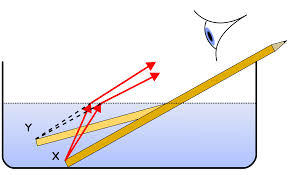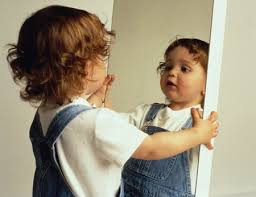Refraction
4 years ago
bhowell
Save
Edit
Host a game
Live GameLive
Homework
Solo Practice
Practice20 QuestionsShow answers
• Question 1
30 seconds
Q. The velocity of light in vacuum is _______ m/s
3x108
3x1012
3x106
3x1010
• Question 2
30 seconds
Q. We can see objects because of_______
reflection
refraction
transmission
diffraction
• Question 3
30 secondsQ. How does light behave as it enters these mediums?
continue its path as straight line.
bends toward the normal
bends away from the normal
reflects
• Question 4
30 seconds
Q. Absolute refractive index of any medium is always _______.
less than 1
greater than 1
1
0
• Question 5
30 seconds
Q. Swimming pools and the ocean look shallower than they really are due to:
reflection
dispersion
refraction
total internal reflection
• Question 6
30 seconds
Q. The formula to calculate the refractive index is
n=cv
n=v/c
n=c/v
v=nc
• Question 7
30 secondsQ. What is this called?
refraction
reflection
reconstroction
retraction
• Question 8
30 secondsQ. According to the Law of reflection, a light ray striking a mirror
continues moving through the mirror in the same direction
moves into the mirror at a slightly different angle
bounces off the mirror toward the direction it came from
bounces off the mirror at the same angle it hits.
• Question 9
120 seconds
Q. A ray of light traveling from air into crown glass strikes the surface at an angle of 30 degrees. What is the angle of refraction? (air n=1, crown glass n=1.532)
39.7 degrees
19.2 degrees
59.1 degrees
12.3 degrees
• Question 10
30 secondsQ. Glass has a _____________ index of refraction than air.
Equal
Greater
Less
Impossible to say
• Question 11
30 secondsQ. The fact the two angles are the same is an example of....
Refraction
Angle of Incidence
Law of reflection
Magnification
• Question 12
30 seconds
Q. Light travels through a liquid at 2.25 EE 8 m/s. What is the index of the liquid?
1.33
1.0
1.523
2.45
• Question 13
120 seconds
Q. What is the index of refraction of a refractive liquid if the angle of incidence in the air is 35 degrees and the angle of refraction is 14 degrees?
1.33
2.21
2.37
1.72
• Question 14
120 seconds
Q. If the angle of incidence of light traveling through air, and then striking water is 30 degrees what is the angle of refraction? (n=1 air, n=1.33 water)
15 degrees
22 degrees
43 degrees
17 degrees
• Question 15
30 seconds
Q. Light has the lowest velocity in which medium?
flint glass n=1.70
barium glass n=1.60
crown glass n=1.523
water n=1.33
diamond n=2.45
diamond
water
flint glass
barium glass
• Question 16
30 secondsQ. The point where the rays meet is known as....
Fire
the focal point
refraction
Plane of the lens
• Question 17
30 seconds
Q. The bouncing of light is known as
Mirror
Refraction
Index of refraction
reflection
• Question 18
30 secondsQ. What is this image showing?
Reflection
Refraction
The law of reflection
Concave Mirror
• Question 19
60 secondsQ. The image formed in a flat mirror is
larger than the object and farther away.
reduced and closer to the mirror.
a real image of the object.
equal to the height and distance of the object.
• Question 20
60 secondsQ. Why does the lower part of the child appear so much different in size from the upper part?